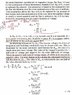# Question on Bmax in magnetics

yungman
I don't understand what the book said. See attached page,

1) The Red underlined section. I don't understand why B will be at it's max negative value at the beginning of the cycle assume it is sine wave start at V=0 at t=0.
2) What is the "subsequent half-cycle? Why is it has to be divided by 2?

3) Notice in the equation, the integration is only for half the cycle from 0 to π/ω? If this is because the result is zero if integrate a full cycle, why is it not having a multiply by 2 in it for the full cycle?

Thanks

#### Attachments

•Bmax L.jpg
47.3 KB · Views: 375

Mentor
I don't understand why B will be at it's max negative value at the beginning of the cycle assume it is sine wave start at V=0 at t=0.
Minimal electric field <=> maximal change in electric field <=> maximal magnetic field <=> minimal change in magnetic field
The sign is arbitrary.

2) What is the "subsequent half-cycle? Why is it has to be divided by 2?
1/2 of the total period. The magnetic field will change from -B_max to +B_max, therefore change by 2 B_max.

why is it not having a multiply by 2 in it for the full cycle?
I don't understand that question.

In general, a bit more context would be useful to understand your problems.

yungman
Thanks for the reply. If you look at the equation, the integration is only for half cycle, so for whole cycle, it should multiply by 2.

The exact words about Bmax is

"When the positive half-cycle of voltage begins, B will be at it's maximum negative value. The total change in flux during the subsequent half-cycle will therefore be 2Bmax"

This is where my confusion. It is not talking about change of magnetic field. Why B at max negative value at the beginning of the cycle when voltage just starting to swing positive from 0V ( assume it is sine wave). Or unless the book use cosine wave! Then voltage is cos 0=1, at it's max at the very beginning of the cycle. Then in the half cycle, cosπ=-1.

Last edited:
Mentor
Thanks for the reply. If you look at the equation, the integration is only for half cycle, so for whole cycle, it should multiply by 2.
Why?
If you travel 10km to the north in 1/2 hour and 10km to the south in the following 1/2 hour, your total change in position is 0km and not 2*10km.

Why B at max negative value at the beginning of the cycle when voltage just starting to swing positive from 0V ( assume it is sine wave). Or unless the book use cosine wave!
Magnetic and electric field have a phase difference of 90° = pi/2. In your example, electric field is a sine wave, so the magnetic field is cosine (with arbitrary signs, as mentioned).

yungman
Why?
If you travel 10km to the north in 1/2 hour and 10km to the south in the following 1/2 hour, your total change in position is 0km and not 2*10km.

Magnetic and electric field have a phase difference of 90° = pi/2. In your example, electric field is a sine wave, so the magnetic field is cosine (with arbitrary signs, as mentioned).

Right, we all know if you integrate a sine a whole cycle, it is zero, that's the reason most integrate half cycle and multiply by 2.

I think I get the second part, as E and B are 90 deg apart, and when a sine wave run 1/2 cycle, the B goes from +ve max to -ve max. But with this idea, if I consider the voltage wave is a cosine wave and swing half cycle, the B will be a sine wave that goes from 0 to max and then back to 0. So the total change is zero!

Mentor
But with this idea, if I consider the voltage wave is a cosine wave and swing half cycle, the B will be a sine wave that goes from 0 to max and then back to 0. So the total change is zero!
The text gives V(t=0)=0, so electric field is a sine wave here.
If you use a different starting point, you are right, but that is not done here.

yungman
Thanks for the help.